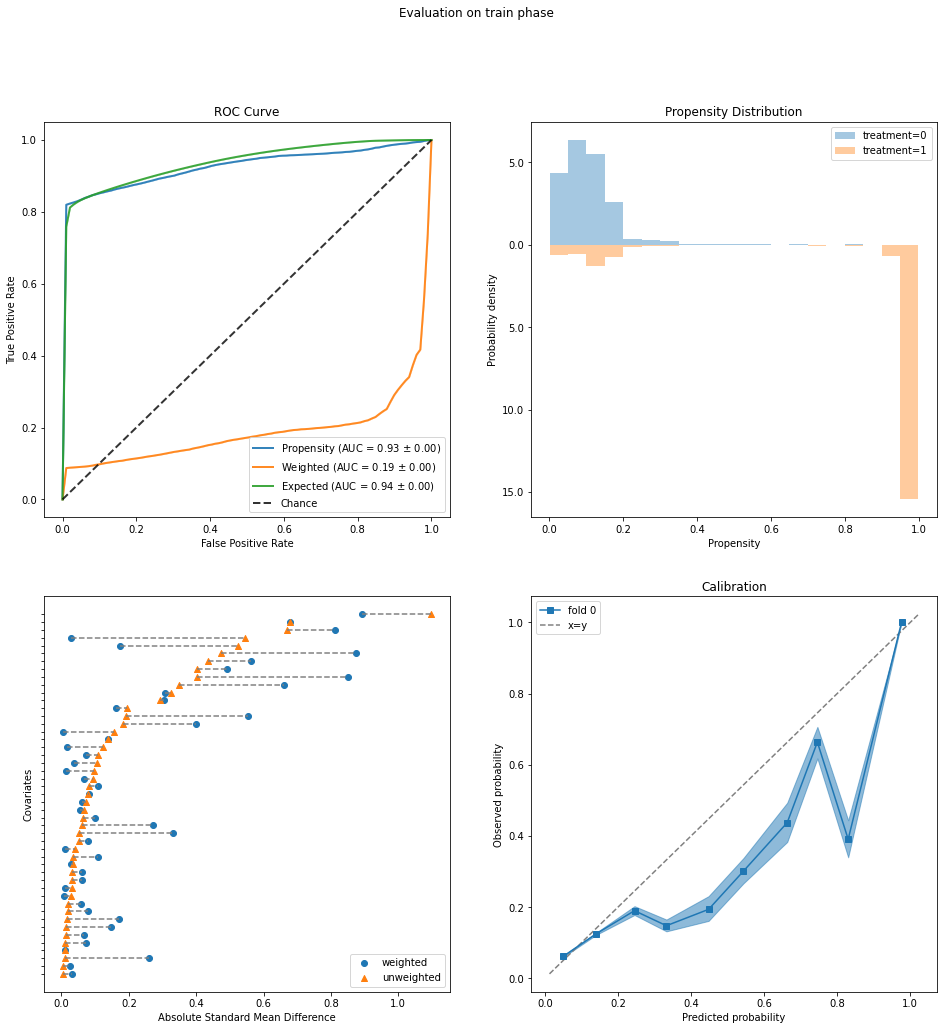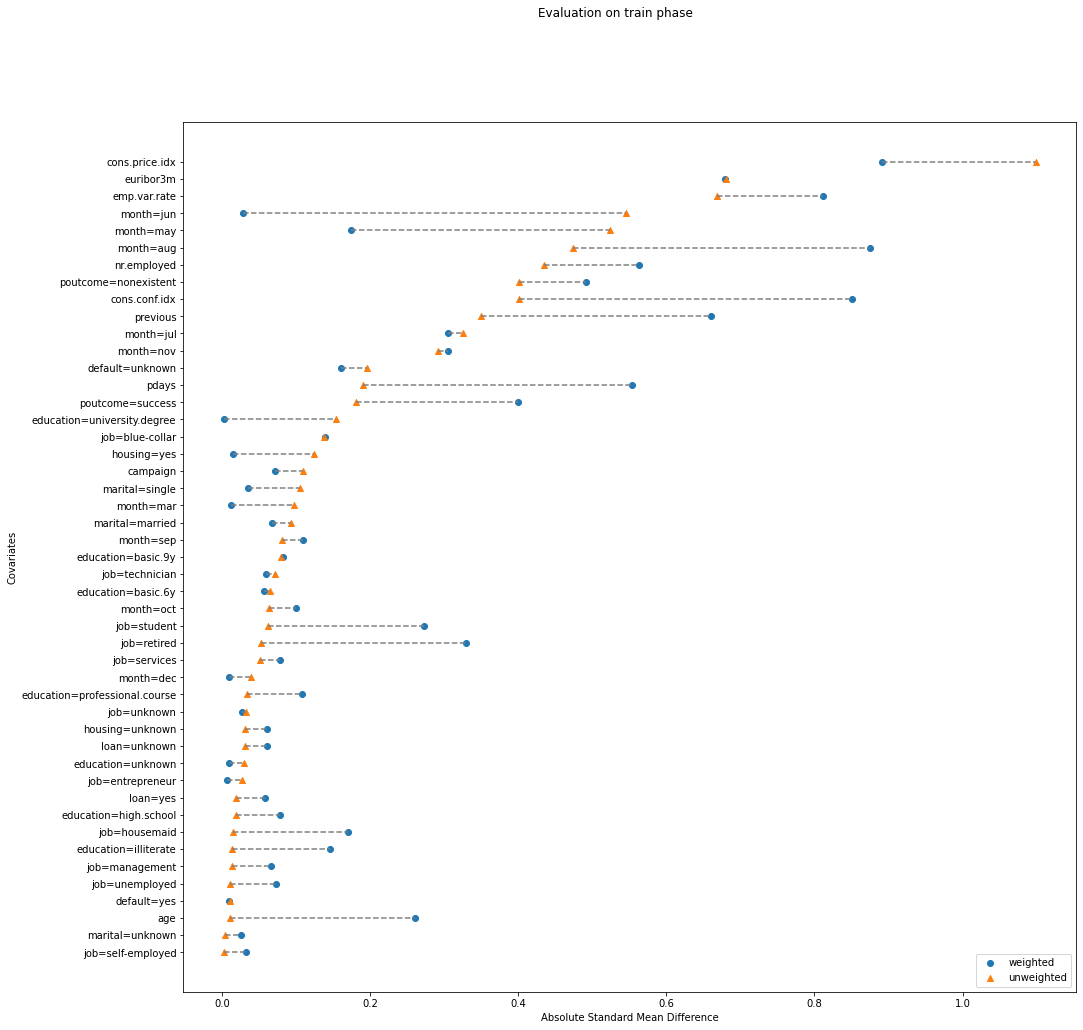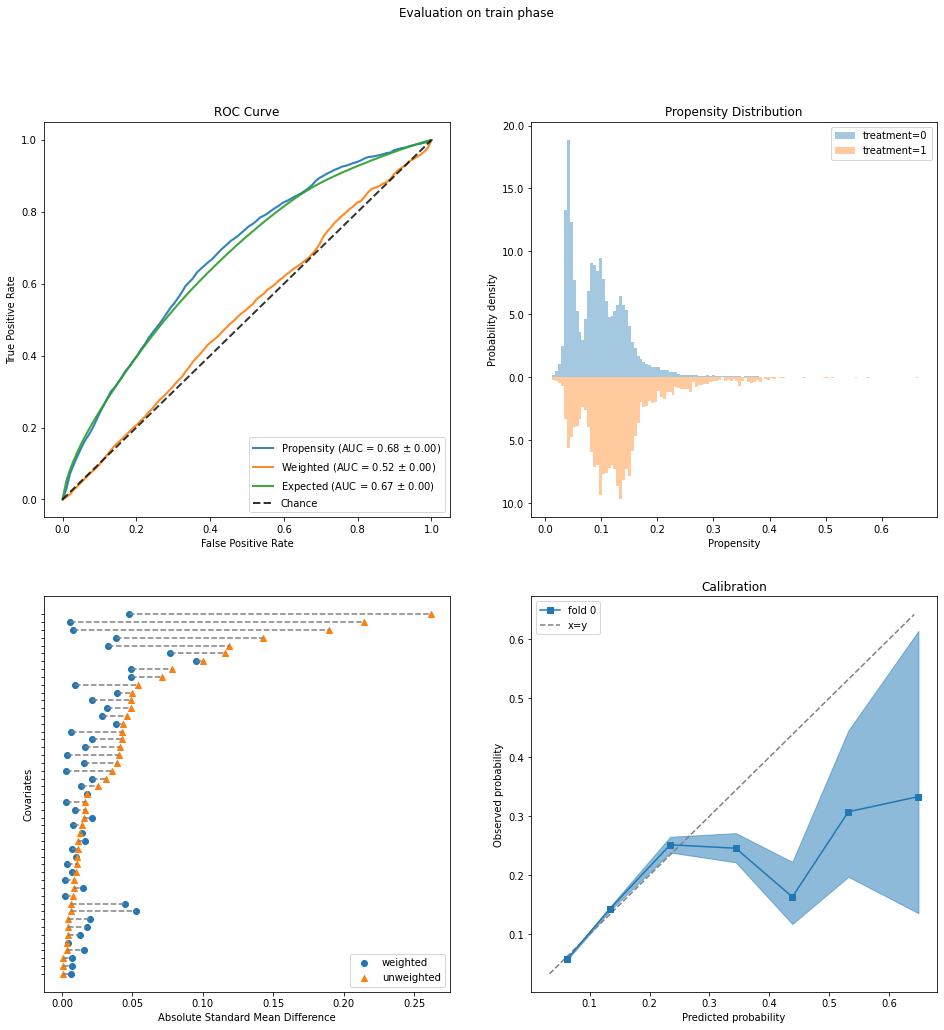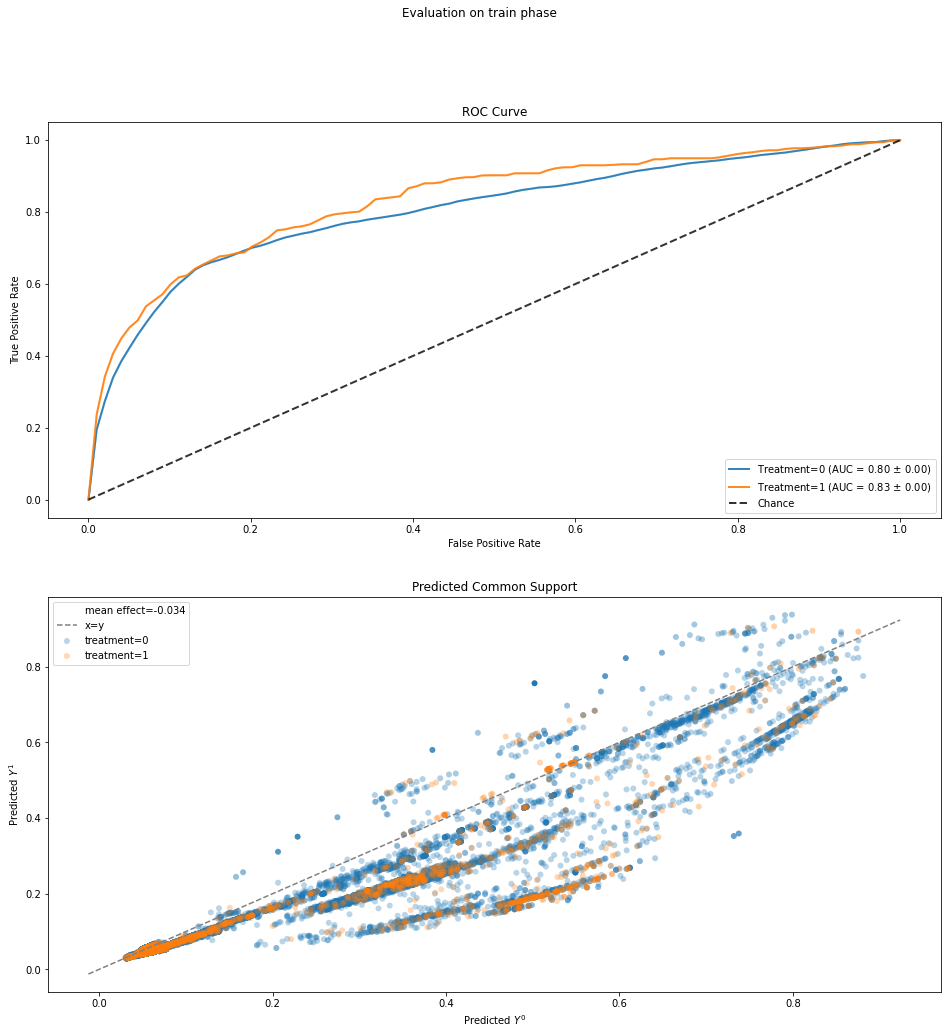# The Effects of Marketing Decisions using the Bank Marketing Dataset¶

The UCI Bank Marketing dataset comes from a real-world bank's direct marketing campaign. It is a popular dataset most commonly treated as a classification task, to predict whether a client will open a term deposit account. In this notebook, we show that it is equally suitable for causal inference. The fraction of clients making term deposits is an outcome that the bank would like to increase, and the dataset contains several variables that could be seen as interventions or treatments (we will use the terms interchangeably) for doing so.

NOTE: Section 2.B uses another package called AIX360 (also created by IBM Research). If you do not wish to install and run AIX360, you can simply skip the first code cell in that section.

## 1. Understand Data¶

In :
import numpy as np
import pandas as pd

"""Reads the bank-marketing data table from a zip file directly from UCI"""
import zipfile
import io
from urllib import request

with request.urlopen(url) as r:
return df

In :
data = read_data_from_UCI()
data.shape

Out:
(41188, 21)

The first step is to understand what variables are present in the data.

In :
data.columns

Out:
Index(['age', 'job', 'marital', 'education', 'default', 'housing', 'loan',
'contact', 'month', 'day_of_week', 'duration', 'campaign', 'pdays',
'previous', 'poutcome', 'emp.var.rate', 'cons.price.idx',
'cons.conf.idx', 'euribor3m', 'nr.employed', 'y'],
dtype='object')

According to the data description:

• The first seven variables ('age'-'loan') relate to the client, including basic credit characteristics ('default', 'housing', 'loan').
• The next four variables relate to the last contact with the client during the current campaign: the mode of communication ('contact', cellular/telephone), date ('month', 'day_of_week'), and duration of the contact ('duration'). 'campaign' is the number of contacts made during this campaign.
• The three subsequent variables relate to previous marketing campaigns, if applicable: the number of days since the last contact from a previous campaign ('pdays'), the number of contacts in previous campaigns ('previous'), and their outcome ('poutcome').
• Variables 'emp.var.rate'-'nr.employed' are economic indicators such as the employment rate and consumer price index.
• The last variable 'y' is the outcome of whether the client opened a term deposit.

We go ahead and binarize 'y', mapping 'yes' to value 1.

In :
print(data['y'].unique())

from sklearn.preprocessing import LabelEncoder
le = LabelEncoder()
y = pd.Series(le.fit_transform(data['y']))
y.mean()

['no' 'yes']

Out:
0.11265417111780131

### Identify treatment variables¶

Next, we consider which of the above variables could be regarded as interventions, undertaken by bank employees, to increase the rate of positive outcomes. These are immediately limited to the variables associated with the current campaign, since client characteristics and economic conditions cannot be controlled by the bank, nor can past events be changed. In addition, as discussed here, 'duration' is not known until the last contact is completed and is mostly determined by the client. Of the remaining variables, in this notebook we will investigate the effects of 'contact' (mode of communication) and 'campaign' (number of contacts). 'day_of_week' can be treated similarly as 'contact', as indicated below.

We consider 'contact' first and encode it as a 0/1-valued variable a (0 for cellular, 1 for telephone):

In :
print(data['contact'].unique())
a = pd.Series(le.fit_transform(data['contact']))
a.mean()

['telephone' 'cellular']

Out:
0.3652520151500437

### Identify potential confounders¶

To estimate causal effects from observational data, we must also identify which of the variables are potential confounders, variables that could affect both the outcome 'y' as well as the decision to intervene. We will want to "adjust for" (i.e. control) these confounders to isolate the causal effect of the intervention on 'y'. For this dataset, confounder selection can be done by:

1. Following the rule of thumb of avoiding post-intervention variables, i.e., those that may be affected by the intervention, and
2. Putting ourselves in the shoes of the hypothetical bank employee who made the decision.

Consideration 1 eliminates 'duration' since it is a result of the last contact with the client. On the flip side, we will always include as potential confounders:

• Client characteristics 'age'-'loan': These clearly affect the client's decision to invest in a term deposit (the outcome). We assume that the bank may also have most of this information in their records and a bank employee may consult it in contacting the client.
• Previous campaigns 'pdays', 'previous', 'poutcome': These indicate the client's previous receptiveness to the bank's products and would also be part of the client's record.
• Economic indicators 'emp.var.rate'-'nr.employed': These conditions may influence the client's decision as well as the bank's practices.
In :
confounders = ['age', 'job', 'marital', 'education', 'default', 'housing', 'loan', 'pdays',
'previous', 'poutcome', 'emp.var.rate', 'cons.price.idx', 'cons.conf.idx', 'euribor3m', 'nr.employed']


For the 'contact' intervention, the decision is whether the bank employee should call a cell phone or landline as the next (and last) contact with the client. Thus we will also include as potential confounders 'month', to account for any seasonality effects, and 'campaign', the number of contacts up until this point.

In :
confounders += ['month', 'campaign']


We will discuss the 'campaign' intervention later below.

Now we just extract the confounders into a variable X and dummy-code (aka one-hot code) the categorical ones in preparation for modelling.

In :
X = data[confounders]
X.dtypes

Out:
age                 int64
job                object
marital            object
education          object
default            object
housing            object
loan               object
pdays               int64
previous            int64
poutcome           object
emp.var.rate      float64
cons.price.idx    float64
cons.conf.idx     float64
euribor3m         float64
nr.employed       float64
month              object
campaign            int64
dtype: object
In :
X = pd.get_dummies(X, prefix_sep='=', drop_first=True)

Out:
age pdays previous emp.var.rate cons.price.idx cons.conf.idx euribor3m nr.employed campaign job=blue-collar ... poutcome=success month=aug month=dec month=jul month=jun month=mar month=may month=nov month=oct month=sep
0 56 999 0 1.1 93.994 -36.4 4.857 5191.0 1 0 ... 0 0 0 0 0 0 1 0 0 0
1 57 999 0 1.1 93.994 -36.4 4.857 5191.0 1 0 ... 0 0 0 0 0 0 1 0 0 0
2 37 999 0 1.1 93.994 -36.4 4.857 5191.0 1 0 ... 0 0 0 0 0 0 1 0 0 0
3 40 999 0 1.1 93.994 -36.4 4.857 5191.0 1 0 ... 0 0 0 0 0 0 1 0 0 0
4 56 999 0 1.1 93.994 -36.4 4.857 5191.0 1 0 ... 0 0 0 0 0 0 1 0 0 0

5 rows × 47 columns

## 2. Effect of Contact Mode¶

We have actually done a good part of the hard work, namely understanding the data and identifying the treatment variables and confounders. Now we can put the tools of Causal Inference 360 to work.

We will first study the causal effect of contact mode 'contact'. Specifically, we will consider the average causal effect, comparing the rate of positive outcomes (term deposits opened) that would result if everyone had been contacted by cell phone versus the rate if everyone had been contacted by landline.

We will estimate the effect in two different ways: inverse propensity weighting (IPW) and standardization. You can look at their respective notebooks (IPW, standardization) for more details on each and also try a third method on your own, namely doubly robust regression. Recall that in causal inference from observational data, the true causal effect is never known: for each client, we observe only one outcome corresponding to the intervention they were assigned, and we cannot go back in time and run a randomized experiment (randomly assigning clients to interventions). By estimating the effect in different ways, relying on different assumptions, we can increase confidence in the results we obtain if they are close to each other.

### Inverse propensity weighting: First attempt¶

Inverse propensity weighting (IPW) requires a model for estimating the probabilities of treatment assignments given the confounders X (known as propensities). Here we will start with plain logistic regression.

In :
from sklearn.linear_model import LogisticRegression
from causallib.estimation import IPW

lr = LogisticRegression(solver='lbfgs', max_iter=1000)
#lr = LogisticRegression(penalty='l1', solver='saga', max_iter=1000)
ipw = IPW(lr)


Fit the model:

In :
ipw.fit(X, a)

Out:
IPW(clip_min=None, clip_max=None, use_stabilized=False,
learner=LogisticRegression(C=1.0, class_weight=None, dual=False, fit_intercept=True,
intercept_scaling=1, max_iter=1000, multi_class='warn',
n_jobs=None, penalty='l2', random_state=None, solver='lbfgs',
tol=0.0001, verbose=0, warm_start=False))

Estimating the rates of positive outcomes under the two interventions (0 if everyone is contacted by cell phone, 1 by landline) is then simply a matter of calling the estimate_population_outcome method of the IPW object.

In :
outcomes = ipw.estimate_population_outcome(X, a, y)
outcomes

Out:
1    0.218004
0    0.155610
dtype: float64

However, before we accept this result, we have to check the IPW model for any problems that we can detect with the observed data. We do this using Causal Inference 360's PropensityEvaluator and ask it to produce four plots.

In :
%matplotlib inline
from causallib.evaluation import PropensityEvaluator

evaluator = PropensityEvaluator(ipw)
eval_results = evaluator.evaluate_simple(X, a, y, plots=["roc_curve", "weight_distribution", "covariate_balance_love", "calibration"])
evaluator.evaluate_simple(X, a, y, plots=["covariate_balance_love"])

Out:
<causallib.evaluation.evaluator.EvaluationResults at 0x158c471d0>The PropensityEvaluator plots show the following problems:

• ROC Curve: The blue curve labelled "Propensity" is the standard ROC for predicting treatment a from the confounders X. In normal classification, we want a high AUC value close to 1, but for causal inference, an AUC that is too high (0.93 here) indicates that the two treatment groups (cellular/telephone) are too easily separated. In particular, the vertical segment at the left end of the ROC indicates that we can correctly identify a large fraction of telephone contacts (true positives) with hardly any cellular contacts mixed in (false positives). In addition, the orange curve labelled "Weighted" should ideally have an AUC of 0.5, meaning that the two treatment groups become indistinguishable after the propensity weighting is applied. The current AUC of 0.19 is way off.
• Propensity Distribution: This plot shows the distributions of propensity values for a=1 (estimated probability of being contacted by telephone) for the two treatment assignment groups: cellular (blue) and telephone (orange). The large orange bars at the right without any blue bars above them again point to the existence of a population that was essentially always contacted by telephone and never by cellular.
• Covariate Balance (bottom left): The orange triangles in this plot (called a Love plot) show that the two treatment groups have large differences in the mean values of some of the covariates (confounders). Re-plotting the Love plot by itself, we see that the largest differences are in the economic indicators 'cons.price.idx', 'euribor3m', 'emp.var.rate'. Furthermore, the propensity weighting has not really succeeded in reducing these differences (blue circles).

Taken together, the PropensityEvaluator shows a lack of overlap between the two treatment groups, and specifically a group of clients that was almost always contacted by telephone. It is not advisable to estimate causal effects in regions where we do not have treatment overlap, unless we are willing to make strong assumptions about how outcomes extrapolate from one region to another (i.e., what would be the outcome under the cellular treatment for the group that was always contacted by telephone).

To proceed, we will exclude this group of telephone-only contacts and try again. The Propensity Distribution plot above suggests that this could be done simply by thresholding the estimated propensity values (at say 0.9). To gain more insight, we will instead use a rule set learner from IBM's AI Explainability 360 (AIX360) toolkit to describe this group by a small number of rules.

### Characterizing the region of treatment non-overlap using rules¶

We use AIX360's Boolean Rules via Column Generation (BRCG) method to learn a set of rules for predicting a=1 (telephone contact) based on the confounders X. To learn more about BRCG, please see the documentation and paper. If you do not wish to install and run AIX360, you can simply skip the following cell. The rules returned by BRCG are hard-coded in the next cell.

In :
from aix360.algorithms.rbm import BooleanRuleCG, FeatureBinarizer
fb = FeatureBinarizer(negations=True)
X_fb = fb.fit_transform(X)
br = BooleanRuleCG()
br.fit(X_fb, a)

from sklearn.metrics import accuracy_score, roc_auc_score
aPred = br.predict(X_fb)
print('Accuracy: {}'.format(accuracy_score(a, aPred)))
print('AUC: {}'.format(roc_auc_score(a, aPred)))
print(br.explain()['rules'])

Learning DNF rule with complexity parameters lambda0=0.001, lambda1=0.001
Initial LP solved
Iteration: 1, Objective: 0.0930
Accuracy: 0.92942119063805
AUC: 0.9033834086679075
['cons.price.idx > 93.92 AND euribor3m > 1.41']


The last line above shows that the returned rule set consists of only a single rule with two conditions on 'cons.price.idx' and 'euribor3m'. This single rule already predicts a very well, as seen from the accuracy and AUC values. Not coincidentally, in the Love plot in the previous subsection, 'cons.price.idx' and 'euribor3m' are also the covariates with the largest mean difference between the treatment groups. We will simply use this rule as the criteria for excluding individuals from the analysis.

In :
indExclude = (X['cons.price.idx'] > 93.92) & (X['euribor3m'] > 1.41)
a[indExclude].mean()

Out:
1.0

We see in fact that in every instance satisfying the rule, the client was contacted by telephone. It is not clear why this is the case without more specific knowledge of the dataset. We can infer that this occurred during a time of higher inflation and interest rates: The consumer price index 'cons.price.idx' is a standard measure of inflation, while Euribor 'euribor3m' is a Eurozone interbank lending rate and a benchmark for short-term interest rates.

Removing instances that satisfy the rule:

In :
y = y.loc[~indExclude]
a = a.loc[~indExclude]
X = X.loc[~indExclude]
print(y.mean())
print(a.mean())

0.14498640322192008
0.1000654022236756


The fraction of telephone contacts has gone down significantly after the exclusion, and the rate of positive outcomes has also increased.

### Inverse propensity weighting: After excluding non-overlap region¶

We now re-fit and re-evaluate the IPW model after removing the non-overlap region identified above.

In :
ipw.fit(X, a)

Out:
IPW(clip_min=None, clip_max=None, use_stabilized=False,
learner=LogisticRegression(C=1.0, class_weight=None, dual=False, fit_intercept=True,
intercept_scaling=1, max_iter=1000, multi_class='warn',
n_jobs=None, penalty='l2', random_state=None, solver='lbfgs',
tol=0.0001, verbose=0, warm_start=False))
In :
evaluator = PropensityEvaluator(ipw)
eval_results = evaluator.evaluate_simple(X, a, y, plots=["roc_curve", "weight_distribution", "covariate_balance_love", "calibration"])The evaluation looks much better.

• ROC Curve: The original AUC of 0.68 ("Propensity", blue curve) is not too high, and the weighted AUC (orange) is close to 0.5.
• Propensity Distribution: No more large orange bars that do not overlap with blue ones.
• Covariate Balance Love Plot: The starting mean differences are now much smaller, below 0.3 on this standardized scale, and are decreased to below 0.1 by propensity weighting.
• Calibration: The calibration curve does deviate significantly from the ideal y=x line. However, this occurs above a predicted probability of 0.3, which the Propensity Distribution plot above shows is very infrequent, so it is not too much of a concern.

Armed with greater confidence from these improved PropensityEvaluator results, we re-estimate the rates of positive outcomes under the two interventions.

In :
outcomes = ipw.estimate_population_outcome(X, a, y)
print(outcomes)
ipw.estimate_effect(outcomes, outcomes, effect_types=['diff','ratio'])

1    0.120666
0    0.150452
dtype: float64

Out:
diff     0.029786
ratio    1.246846
dtype: float64

We conclude from the IPW analysis that contacting clients by cell phone results in a higher rate of opening term deposits than by landline, 15% to 12%, a difference of 3.0% and a relative increase of almost 25%.

### Standardization¶

Next we turn to standardization as a second method for estimating the causal effect of contact mode. We will continue to exclude the telephone-only group identified above to avoid having to extrapolate.

Standardization requires a model for estimating the outcome y given intervention a and confounders X. We will use gradient-boosted classification trees as well as the predict_proba=True option to focus on predicting probabilities of outcomes.

In :
from sklearn.ensemble import GradientBoostingClassifier
from causallib.estimation import Standardization

#gb = LinearRegression()
std = Standardization(gb, predict_proba=True)
std.fit(X, a, y)

Out:
Standardization(encode_treatment=False, predict_proba=True,
learning_rate=0.1, loss='deviance', max_depth=3,
max_features=None, max_leaf_nodes=None,
min_impurity_decrease=0.0, min_impurity_split=None,
min_samples_leaf=1, min_samples_split=2,
min_weight_fraction_leaf=0.0, n_estimators=100,
n_iter_no_change=None, presort='auto', random_state=None,
subsample=1.0, tol=0.0001, validation_fraction=0.1,
verbose=0, warm_start=False))
In :
outcomes = std.estimate_population_outcome(X, a).xs(1, level='y')
print(outcomes)
std.estimate_effect(outcomes, outcomes, effect_types=['diff','ratio'])

a
0    0.150324
1    0.116808
dtype: float64

Out:
diff     0.033516
ratio    1.286933
dtype: float64

These values are similar to the ones obtained with IPW: an absolute increase of 3.4% in positive outcomes with cellular contact compared to landline, and a relative increase of 29%.

Causal Inference 360 provides an OutcomeEvaluator class to evaluate standardization models, similar to PropensityEvaluator for propensity weighting models. We apply OutcomeEvaluator below.

In :
import warnings
warnings.filterwarnings('ignore')

from causallib.evaluation import OutcomeEvaluator
evaluator = OutcomeEvaluator(std)
eval_results = evaluator.evaluate_simple(X, a, y, plots=["roc_curve","common_support"])• ROC Curve: The AUC in predicting outcome y is reasonably high and similar for both treatment groups.
• Predicted Common Support: This plot is meant to assess the ignorability assumption, which states that the counterfactual outcomes (Y0, Y1) are independent of the actual treatment assignment when conditioned on the confounders. The plot shows the distributions of predicted (Y0, Y1) values and we are looking for good overlap between the distributions for treatment groups 0 (blue) and 1 (orange). This appears to be the case.

The Standardization class uses a single regressor that takes input (X, a) to predict y, i.e., the intervention a is placed on the same footing as other input features in X. Alternatively, StratifiedStandardization uses two regressors, one for a=0 and one for a=1, each of which take X as input.

In :
from causallib.estimation import StratifiedStandardization
std = StratifiedStandardization(gb, predict_proba=True)
std.fit(X, a, y)

outcomes = std.estimate_population_outcome(X, a).xs(1, level='y')
print(outcomes)
std.estimate_effect(outcomes, outcomes, effect_types=['diff','ratio'])

a
0    0.150330
1    0.113162
dtype: float64

Out:
diff     0.037168
ratio    1.328444
dtype: float64

The resulting estimates are a bit higher than those from Standardization.

In :
evaluator = OutcomeEvaluator(std)
eval_results = evaluator.evaluate_simple(X, a, y, plots=["roc_curve","common_support"])